# Fefferman-Garsia inequality

(diff) ← Older revision | Latest revision (diff) | Newer revision → (diff)

C. Fefferman [a3] discovered the remarkable fact that the space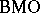is none other than the "dual" of the Hardy spacein the sense of function analysis (cf. also Functional analysis; Hardy spaces; Duality;-space). In establishing the above duality, Fefferman discovered the following "formal" inequality: if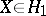and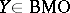, then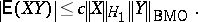The word "formal" is used here sincedoes not necessarily have a finite Lebesgue integral. However, one can defineby setting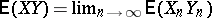, since it has been proved that in this caseexists. Here,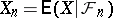and,,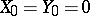a.s., are regular martingales. Later, A.M. Garsia [a4] proved an analogous inequality forwith.

S. Ishak and J. Mogyorodi [a5] extended the validity of the Fefferman–Garsia inequality to all. In 1983, [a6], [a7], [a8], they also proved the following generalization: If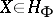and, where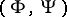is a pair of conjugate Young functions (cf. also Dual functions) such thathas a finite power, then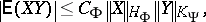where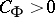is a constant depending only onandstands for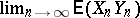, which exists.

It was proved in [a1], [a2] that the generalized Fefferman–Garsia inequality holds if and only if the right-hand side of the corresponding Burkholder–Davis–Gundy inequality holds.

How to Cite This Entry:
Fefferman-Garsia inequality. Encyclopedia of Mathematics. URL: http://encyclopediaofmath.org/index.php?title=Fefferman-Garsia_inequality&oldid=17021
This article was adapted from an original article by N.L. Bassily (originator), which appeared in Encyclopedia of Mathematics - ISBN 1402006098. See original article